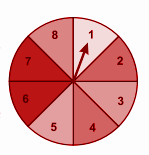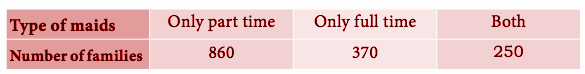# Samacheer Kalvi 9th Maths Book Solutions Chapter 9 | Probability Answers For Tamil Nadu Board

Samacheer Kalvi 9th Maths Book Solutions Chapter 9 – Probability is available here. The Samacheer Kalvi 9th Maths book answers of Chapter 9, available at BYJU’S, contain step by step explanations designed by our Mathematics experts. All these important questions are based on the new pattern prescribed by the Tamil Nadu board. Students can also get the solutions of other chapters on Samacheer Kalvi 9th Maths solutions. Students are advised to refer to these Samacheer Kalvi Class 9 Maths Book Chapter 9 Questions and Solutions for practice.

## Samacheer Kalvi Class 9 Maths Book Chapter 9 Questions with Solutions

Chapter 9 of the Samacheer Kalvi 9th Maths guide will help the students to solve problems related to the classical approach, empirical approach, types of events.

### Samacheer Kalvi 9th Maths Chapter 9: Probability Book Exercise 9.1 Questions and Solutions

Question 1: You are walking along a street. If you just choose a stranger crossing you, what is the probability that his next birthday will fall on a Sunday?

Solution:

Number of days = S = {Monday, Tuesday, Wednesday, Thursday, Friday, Saturday, Sunday}

n (S) = 7

Let A be the event of being birthday on Sunday.

n(A) = 1

P(A) = n(A) / n(S)

P(A) = 1 / 7

Question 2: What is the probability of drawing a King or a Queen or a Jack from a deck of cards?

Solution:

Total number of cards n(S) = 52

Let A be the event of getting a king or queen or jack card.

n(A) = 4 + 4 + 4 = 12

P(A) = n(A) / n(S)

P(A) = 12 / 52

P(A) = 3 / 13

Question 3: What is the probability of throwing an even number with a single standard dice of six faces?

Solution:

Sample space = {1, 2, 3, 4, 5, 6}

n(S) = 6

Let A be the event of getting an even number.

A = {2, 4, 6}

n(A) = 3

P(A) = n(A) / n(S)

= 3 / 6 = 1 / 2

Question 4: There are 24 balls in a pot. If 3 of them are red, 5 of them are blue and the remaining are green then, what is the probability of picking out

(i) a blue ball

(ii) a red ball

(iii) a green ball

Solution:

The number of balls n(S) = 24

Let A, B and C be the events of getting red, blue and green balls, respectively.

n(A) = 3, n(B) = 5, n(C) = 24 – (5 + 3) = 16

(i) p(A) = n(A) / n(S)

P(A) = 3 / 24

P(A) = 1 / 8

(ii) P(B) = n(B) / n(S)

P(B) = 5 / 24

(iii) P(C) = n(C) / n(S)

P(C) = 16 / 24

P(C) = 2 / 3

Question 5: When two coins are tossed, what is the probability that two heads are obtained?

Solution:

Outcomes of tossing two coins

S = { HH, TT, HT, TH}

n(S) = 4

Let “A” be the event of getting 2 heads.

A = {HH}

n(A) = 1

P(A) = n(A) / n(S)

P(A) = ¼

Question 6: Two dice are rolled, find the probability that the sum is

(i) equal to 1

(ii) equal to 4

(iii) less than 13

Solution:

The sample space S of two dice is given below.

S = { (1,1),(1,2),(1,3),(1,4),(1,5),(1,6)

(2,1),(2,2),(2,3),(2,4),(2,5),(2,6)

(3,1),(3,2),(3,3),(3,4),(3,5),(3,6)

(4,1),(4,2),(4,3),(4,4),(4,5),(4,6)

(5,1),(5,2),(5,3),(5,4),(5,5),(5,6)

(6,1),(6,2),(6,3),(6,4),(6,5),(6,6) }

Let E be the event sum equal to 1. There are 0 outcomes which correspond to a sum equal to 1.

P(E) = n(E) / n(S) = 0 / 36 = 0

(b) Three possible outcomes give a sum equal to 4.

E = {(1,3),(2,2),(3,1)},

P(E) = n(E) / n(S) = 3 / 36 = 1 / 12

(c) All possible outcomes, E = S, give a sum less than 13.

P(E) = n(E) / n(S) = 36 / 36 = 1

Question 7: A manufacturer tested 7000 LED lights at random and found that 25 of them were defective. If a LED light is selected at random, what is the probability that the selected LED light is a defective one?

Solution:

Total number of outcomes = 7000

Number of non defective bulbs = 7000 – 25 = 6975

P (of getting a non-defective bulb) = 6975 / 7000 = 279 / 280

P (of getting a defective bulb) = 25 / 7000 = 1 / 280

Question 8: In a football match, a goalkeeper of a team can stop the goal, 32 times out of 40 attempts tried by a team. Find the probability that the opponent team can convert

the attempt into a goal.

Solution:

Number of attempts = 40

Goals blocked = 32

Goals scored = 8

The probability that a goal will be scored

= Favourable outcome / Total number of outcomes

= 8 / 40

= 1 / 5 or 0.2

Question 9: What is the probability that the spinner will not land on a multiple of 3?

Solution:

Let E be the event that the spinner will not land on a multiple of 3.

Total number of outcome = 8

Favourable outcome = 6

P (E) = 6 / 8

= 3 / 4

Question 10: Frame two problems in calculating probability, based on the spinner shown here.Solution:

What is the probability of getting 8 on spinner?

What is the probability of getting factors of 24?

### Samacheer Kalvi 9th Maths Chapter 9: Probability Book Exercise 9.2 Questions and Solutions

Question 1: A company manufactures 10000 Laptops in 6 months. Out of which 25 of them are found to be defective. When you choose one Laptop from the manufacturer, what is the probability that the selected Laptop is a good one?

Solution:

Number of laptops n(S) = 10000

Number of defective laptops = 25

Let A be the event of choosing a good laptop.

Number of good laptop = 10000 – 25

n(A) = 9975

P(A) = n(A) / n(S)

P(A) = 9975 / 10000

P(A) = 0.9975

Question 2: In a survey of 400 youngsters aged 16-20 years, it was found that 191 have their voter ID card. If a youngster is selected at random, find the probability that the youngster does not have their voter ID card.

Solution:

Total number of youngster n(S) = 400

Let A be the event of choosing youngster does not have an ID card

n(A) = 400 – 191

n(A) = 209

P(A) = n(A) / n(S)

P(A) = 209 / 400

P(A) = 0.5225

Question 3: The probability of guessing the correct answer to a certain question is x / 3. If the probability of not guessing the correct answer is x / 5, then find the value of x.

Solution:

P(A) = Probability of guessing the correct answer

P(B) = Probability of not guessing the correct answer

P(A) + P(B) = 1

(x / 3) + (x / 5) = 1

(5x + 3x) / 15 = 1

8x = 15

x = 15 / 8

Question 4: If the probability of a player winning a particular tennis match is 0.72. What is the probability of the player losing the match?

Solution:

Let A be the event of winning a game.

Let A bar be the event of losing the game.

P(A) + P(A bar) = 1

0.72 + P(A bar) = 1

P(A bar) = 1 – 0.72

P(A bar) = 0.28

Question 5: 1500 families were surveyed and the following data was recorded about their maids at homesA family is selected at random. Find the probability that the family selected has

(i) Both types of maids

(ii) Part-time maids

(iii) No maids

Solution:

Total number of families n(S) = 1500

Let A be the event of selecting maid of both types.

n(A) = 250

P(A) = n(A) / n(S)

P(A) = 250 / 1500

P(A) = 1 / 6

(ii) Part-time maids

Let B be the event of selecting part time maid

n(B) = 8600

P(B) = n(B) / n(S)

P(B) = 860 / 1500

P(B) = 43 / 75

(iii) No maids

Number of families selected maid = 860 + 370 + 250 = 1480

n(C) = 1500 – 1480

n(C) = 20

P(C) = n(C) / n(S)

= 20 / 1500

P(C) = 1 / 75